Study Materials

# NCERT Solutions for Class 6th Mathematics

Page 1 of 6

## Chapter 4. Basic Geometrical Ideas

### Exercise 4.1

Exercise 4.1

Q1. Use the figure to name: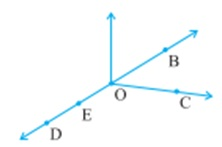(a) Five points

Solution: The five point of this figure:- B, C, O, E, D

(b) A line

Solution: DB

(c) Four rays

Solution: OB, OC, OP, OD

(d) Five line segments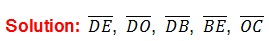Q2. Name the line given in all possible (twelve) ways, choosing only two letters at a time from the four given.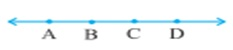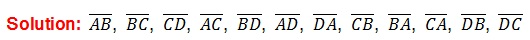Q3. Use the figure to name :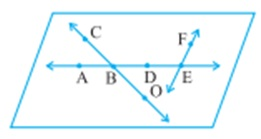Q4. How many lines can pass through

(a) one given point?

Solution: Infinity lines can pass through one point.

(b) Two given points?

Solution: Only one lines can pass through one point.

Q5. Draw a rough figure and label suitably in each of the following cases:.

(a) Point P lies on AB.

Solution: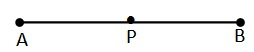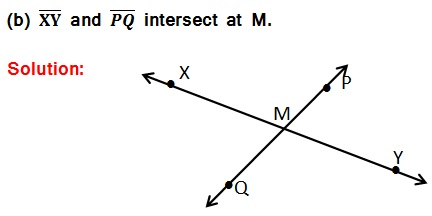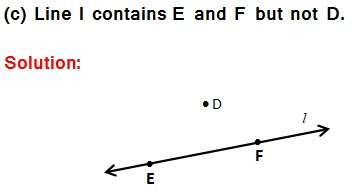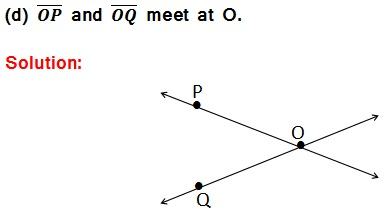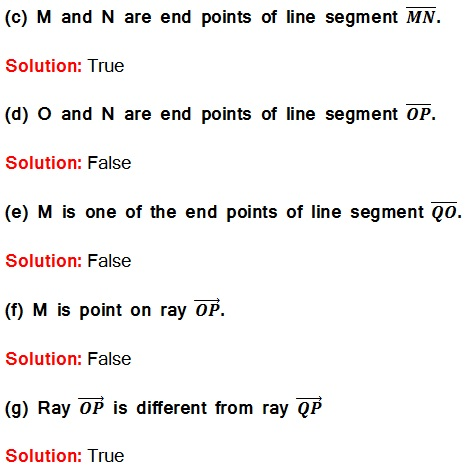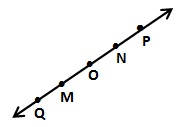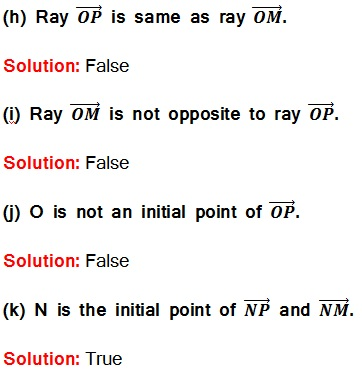Page 1 of 6

Chapter Contents: